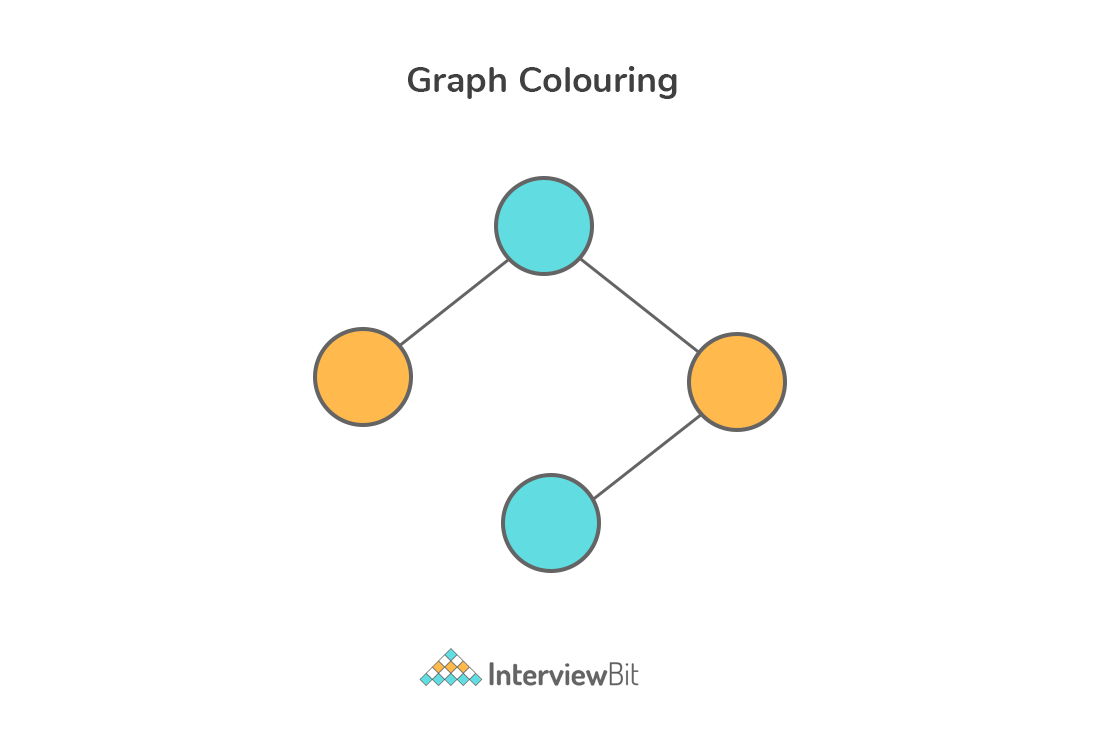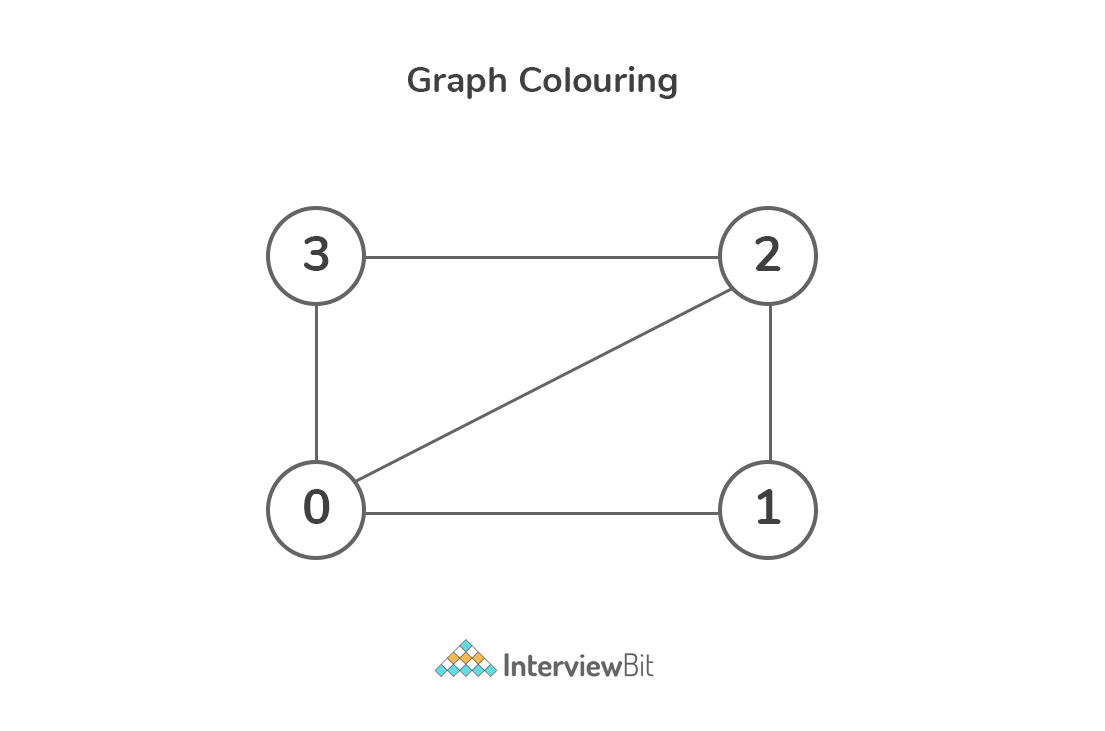Practice
Resources
Contests
Online IDE
New
Free Mock
Scaler
Practice
Improve your coding skills with our resources
Contests
Compete in popular contests with top coders
Scaler
Explore Offerings by SCALER## Welcome to Interviewbit, help us create the best experience for you!

Currently, You are a:College/University *
Enter the name of your college
Branch *
Year of completion *College/University *
Enter the name of your college
Branch *
Year of completion *Current Company *
Enter company name
Experience *## You're all set!Full name *
Email *

By creating an account, I acknowledge that I have read and agree to InterviewBit’s Terms and Privacy Policy .## Welcome back!

Email *Go to Problems

Be a Code Ninja!

# Graph Coloring Algorithm using Backtracking

### What is graph coloring problem?

Graph coloring problem involves assigning colors to certain elements of a graph subject to certain restrictions and constraints. This has found applications in numerous fields in computer science. For example:

• Sudoku: This game is a variation of Graph coloring problem where every cell denotes a node (or vertex) and there exists an edge between two nodes if the nodes are in same row or same column or same block.
• Geographical maps: There can be cases when no two adjacent cities/states can be assigned same color in the maps of countries or states. In this case, only four colors would be sufficient to color any map.

Vertex coloring is the most commonly encountered graph coloring problem. The problem states that given `m` colors, determine a way of coloring the vertices of a graph such that no two adjacent vertices are assigned same color.

`Note: The smallest number of colors needed to color a graph G is called its chromatic number.For example, the following undirected graph can be colored using minimum of 2 colors.Hence the chromatic number of the graph is 2. `### Analysis

• Input:
• A graph represented in 2D array format of size `V * V` where V is the number of vertices in graph and the 2D array is the adjacency matrix representation and value `graph[i][j]` is 1 if there is a direct edge from i to j, otherwise the value is 0.
• An integer `m` that denotes the maximum number of colors which can be used in graph coloring.
• Consider the input as shown in the image below:• The above graph can be represented as follows:
``````graph = {
{ 0, 1, 1, 1 },
{ 1, 0, 1, 0 },
{ 1, 1, 0, 1 },
{ 1, 0, 1, 0 },
};
``````
• Consider `m = 3`.
• Output:
• Return array color of size `V` that has numbers from 1 to m. Note that `color[i]` represents the color assigned to the ith vertex.
• Return `false` if the graph cannot be colored with `m` colors.
• Solution:
• Naive Approach:
• The brute force approach would be to generate all possible combinations (or configurations) of colors.
• After generating a configuration, check if the adjacent vertices have the same colour or not. If the conditions are met, add the combination to the result and break the loop.
• Since each node can be colored by using any of the `m` colors, the total number of possible color configurations are mV. The complexity is exponential which is very huge.
• Pseudo Code:
``````public class InterviewBit{
private static boolean isSafeToColor(int[][] graph, int[] color)
{
// check for every edge, if any adjacent edge has same color, return false
for (int i = 0; i < V; i++)
for (int j = i + 1; j < V; j++)
if (graph[i][j] == 1 && color[j] == color[i])
return false;
return true;
}

private static void printColorArray(int[] color){
System.out.println("Solution colors are: ")
for(int i =0;i < color.length; i++){
System.out.println(color[i]);
}
}

/**
* returns false if the m colors cannot be assigned, else,
* return true and print assignments of colors to all vertices.
* Note: There may be more than one solutions
* The function prints only one of the solutions.
* */
private static boolean graphColoring(int[][] graph, int m, int i, int[] color)
{
// if current index becomes end
if (i == V) {
// check whether its safe to color
if (isSafeToColor(graph, color)) {
//If safe, print solution array and return true
printColorArray(color);
return true;
}
return false;
}

// Assign each color from 1 to m
for (int j = 1; j <= m; j++) {
color[i] = j;

// Check for the rest vertices by recursion
if (graphColoring(graph, m, i + 1, color))
return true;

color[i] = 0;
}

return false;
}
}
``````
• Time Complexity: O(mV).
• Space Complexity: O(V) which is for storing the output array.
• Using Backtracking:
• By using the backtracking method, the main idea is to assign colors one by one to different vertices right from the first vertex (vertex 0).
• Before color assignment, check if the adjacent vertices have same or different color by considering already assigned colors to the adjacent vertices.
• If the color assignment does not violate any constraints, then we mark that color as part of the result. If color assignment is not possible then backtrack and return false.
• Java solution:
``````    public class InterviewBit {
final int V = 4;
int[] color;

boolean isSafeToColor(int v, int[][] graphMatrix, int[] color, int c)
{
//check for each edge
for (int i = 0; i < V; i++)
if (graphMatrix[v][i] == 1 && c == color[i])
return false;
return true;
}

boolean graphColorUtil(int[][] graphMatrix, int m, int[] color, int v)
{
// If all vertices are assigned a color then return true
if (v == V)
return true;

// Try different colors for vertex V
for (int i = 1;i <= m; i++)
{
// check for assignment safety
if (isSafeToColor(v, graphMatrix, color, i))
{
color[v] =i;
// recursion for checking other vertices
if (graphColorUtil(graphMatrix, m, color, v + 1))
return true;
// if color doesnt lead to solution
color[v] = 0;
}
}
// If no color can be assigned to  vertex
return false;
}

void printColoringSolution(int color[])
{
System.out.println("Color schema for vertices are: ");
for (int i = 0; i < V; i++)
System.out.println(color[i]);
}

/**
* It returns false if the m colors cannot be assigned
* otherwise return true and
* print color assignment result to all vertices.
*/
boolean graphColoring(int[][] graphMatrix, int m)
{
// Initialize all color values as 0.
color = new int[V];
Arrays.fill(color,0);

// Call graphColorUtil() for vertex 0
if (!graphColorUtil(graphMatrix, m, color, 0))
{
System.out.println(
"Color schema not possible");
return false;
}

// Print the color schema of vertices
printColoringSolution(color);
return true;
}

// Main driver program
public static void main(String args[])
{
InterviewBit interviewBit
= new InterviewBit();

int graphMatrix[][] = {
{ 0, 1, 1, 1 },
{ 1, 0, 1, 0 },
{ 1, 1, 0, 1 },
{ 1, 0, 1, 0 },
};
int m = 3; // Number of colors
interviewBit.graphColoring(graphMatrix, m);
}
}
``````
• Time ComplexityO(mV). Since backtracking is also a kind of brute force approach, there would be total O(mV) possible color combinations. It is to be noted that the upperbound time complexity remains the same but the average time taken will be less due to the refined approach.
• Space ComplexityO(V) for storing the output array in O(V) space

## Serious about Learning Programming ?

Learn this and a lot more with Scaler Academy's industry vetted curriculum which covers Data Structures & Algorithms in depth.
Examples

## Backtracking Problems

Maths and backtracking
Problem Score Companies Time Status
Maximal String 200
62:53
Kth Permutation Sequence 350 78:06
Gray Code 350
58:37
Subsets
Problem Score Companies Time Status
Subset 250 61:56
Combination Sum 300 62:41
Combination Sum II 300 41:57
Combinations 300 46:09
Subsets II 300 41:13
Bruteforce builder
Problem Score Companies Time Status
Letter Phone 250 53:36
Palindrome Partitioning 300 68:07
Generate all Parentheses II 350
52:54
Permutations
Problem Score Companies Time Status
Permutations 350 39:38
Game solving
Problem Score Companies Time Status
NQueens 550 74:37
Sudoku 700 70:09

Problem Score Companies Time Status
All Possible Combinations 200 23:41Free Mock Assessment
Help us know you better for the best experience
Current Employer *
Enter company name